## CircumcircleA Triangle's circumscribed circle. Its center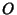is called the Circumcenter, and its Radiusthe Circumradius. The circumcircle can be specified using Trilinear Coordinates as(1)

The Steiner Pointand Tarry Pointlie on the circumcircle.

A Geometric Construction for the circumcircle is given by Pedoe (1995, pp. xii-xiii). The equation for the circumcircle of the Triangle with Verticesfor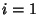, 2, 3 is(2)

Expanding the Determinant,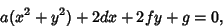(3)

where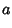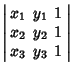(4)(5)(6)(7)

Completing the Square gives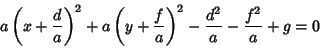(8)

which is a Circle of the form(9)

with Circumcenter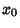(10)(11)(12)